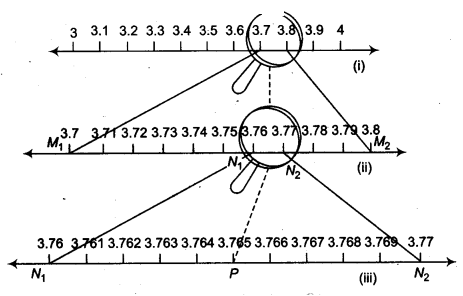NCERT Solutions for Class 9 Maths Chapter 1 Number Systems Ex 1.4 are part of NCERT Solutions for Class 9 Maths. Here we have given NCERT Solutions for Class 9 Maths Chapter 1 Number Systems Ex 1.4.

 Board CBSE Textbook NCERT Class Class 9 Subject Maths Chapter Chapter 1 Chapter Name Number Systems Exercise Ex 1.4 Number of Questions Solved 2 Category NCERT Solutions

## NCERT Solutions for Class 9 Maths Chapter 1 Number Systems Ex 1.4

Question 1.
Visualise 3.765 on the number line, using successive magnification.
Solution:
We know that, 3.765 lies between 3 and 4. So, let us divide the part of the number line between 3 and 4 into 10 equal parts and look at the portion between 3.7 and 3.8 through a magnifying glass. Now 3.765 lies between 3.7 and 3.8 [Fig. (i)]. Now, we imagine to divide this again into ten equal parts. The first mark will represent 3.71, the next 3.72 and soon. To see this clearly,we magnify this as shown in [Fig. (ii)].

Again 3.765 lies between 3.76 and 3.77 [Fig. (ii)]. So, let us focus on this portion of the number line [Fig. (iii)] and imagine to divide it again into ten equal parts [Fig. (iii)]. Here, we can visualise that 3.761 is the first mark and 3.765 is the 5th mark .in these subdivisions. We call this process of visualisation of representation of numbers on the number line through a magnifying glass as the process of successive magnification.

So, we get seen that it is possible by sufficient successive magnifications of visualise the position (or representation) of a real number with a terminating decimal expansion on the number line.Question 2.
Visualise 4.$$\bar { 26 }$$ on the number line, upto 4 decimal places.
Solution:
We adopt process by successive magnification and successively decreasethe lengths of the portion of the number line in which 4.$$\bar { 26 }$$ is located. Since 4.$$\bar { 26 }$$ is located between 4 and 5 and is divided into 10 equal parts [Fig. (i)]. In further, we locate 4.26between 4.2 and 4.3 [Fig. (ii)].

To get more accurate visualisation of the representation, we divide this portion into 10 equal parts and use a magnifying glass to visualise that 4.$$\bar { 26 }$$ lies between 4.26 and 4.27. To visualise 4.$$\bar { 26 }$$ more clearly we divide again between 4.26 and 4.27 into 10 equal parts and visualise the repsentation of 4.$$\bar { 26 }$$ between 4.262 and 4.263 [Fig. (iii)].

Now, for a much better visualisation between 4.262 and 4.263 is agin divided into 10 equal parts [Fig. (iv)]. Notice that 4.$$\bar { 26 }$$ is located closer to 4.263 then to 4.262 at 4.2627.Remark: We can adopt the process endlessly in this manner and simultaneously imagining the decrease in the length of the number line in which 4.$$\bar { 26 }$$ is located.

We hope the NCERT Solutions for Class 9 Maths Chapter 1 Number Systems Ex 1.4, help you. If you have any query regarding NCERT Solutions for Class 9 Maths Chapter 1 Number Systems Ex 1.4, drop a comment below and we will get back to you at the earliest.